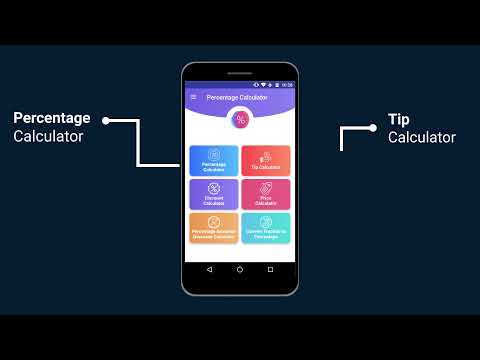# Percentage Calculator -Calculate Percentage EasilyEveryone
37
Offers in-app purchasesPercent calculator is one of the fastest percentage calculator app designed with simplicity and performs various calculations instantly. Do you face difficulties while calculating simple percentage? No need to worry more! Just enter any two values and the third one is computed. With this Percent calculator app you can calculate tip percentage, although just enter your sale price and calculate your discount percentage. You can compare products with various quantities and prices and calculate percentage of price/quantity. Percentage calculator allows you to calculate percentage increase or decrease from a difference of two different values. You can easily convert proper and improper fractions to percentages through this percent calculator also it determines the percent value for a fraction. Thus, you can perform various calculation in just a couple of seconds through this percentage calculator app.

Percent calculator includes:
• Simple Percentage Calculator
• Tip Calculator
• Discount Calculator
• Price/Quantity Calculator
• Percentage increase calculator
• Percent decrease calculator
• Percent change calculator
• Percent difference calculator
• Convert Fraction to Percentage
Collapse

Review Policy
4.2
37 total
5
4
3
2
1

## What's New

Most Powerful Percentage Calculator app
Collapse

Updated
July 13, 2019
Size
5.9M
Installs
1,000+
Current Version
1.7
Requires Android
4.4 and up
Content Rating
Everyone
In-app Products
\$1.99 per item
Permissions
Offered By
EclixTech
Developer